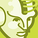# QlikView App Dev

Discussion Board for collaboration related to QlikView App Development.

Announcements
Read about the latest Qlik Community enhancements on the Community News blog!
cancel
Showing results for
Did you mean:Contributor

## Conditional color for Calculated dimension

Hi All,

i have Calculated Dimension like Below:

=Num(Aggr(Count(DISTINCT{<Bucket={On_Time}>}Employee_Number)/Sum(Aggr(Count(DISTINCT Employee_Number), Org_Hier.ORGANIZATION, Bucket, MonthBucket)), MonthBucket),'#,##0.00%')

i want to show Conditional color based on above dimension.

Example:

If (Compliance %) is   <=90   Red

>=91 and <=94   Yellow

95 and above Green

I have tried below expression but not working.

=if(num(Aggr(sum(aggr(Count(DISTINCT{<Status={Ontime}>}Employee_Number),[Updated Location],Region_Name,Site_Name, Status,Complaince_Training_Program, MonthBucket))/
Sum(Aggr(Count(DISTINCT Employee_Number),[Updated Location],Region_Name,Site_Name, Status,Complaince_Training_Program, MonthBucket)), MonthBucket),'##,#0%') <=90,RGB(255,0,0),Yellow())

How to achieve in Calculated Dimension,

Any Help would be greatly appreciated.

Thanks

Joshi

5 RepliesMVP

May be try this

If(Aggr(Count(DISTINCT{<Bucket={On_Time}>}Employee_Number)/Sum(Aggr(Count(DISTINCT Employee_Number), Org_Hier.ORGANIZATION, Bucket, MonthBucket)), MonthBucket) <= 0.90, RGB(255,0,0),

If(Aggr(Count(DISTINCT{<Bucket={On_Time}>}Employee_Number)/Sum(Aggr(Count(DISTINCT Employee_Number), Org_Hier.ORGANIZATION, Bucket, MonthBucket)), MonthBucket) <= 0.94, Yellow(), Green()))Contributor
Author

Hi Sunny,

No Luck only else part showing Green Color.

Thanks,MVP

Can you try this?

If(Count(DISTINCT{<Bucket={On_Time}>}Employee_Number)/Sum(Aggr(Count(DISTINCT Employee_Number), Org_Hier.ORGANIZATION, Bucket, MonthBucket)) <= 0.90, RGB(255,0,0),

If(Count(DISTINCT{<Bucket={On_Time}>}Employee_Number)/Sum(Aggr(Count(DISTINCT Employee_Number), Org_Hier.ORGANIZATION, Bucket, MonthBucket)) <= 0.94, Yellow(), Green()))Contributor
Author

Hi Sunny,

Still no Luck

Thanks,

joshiMVP

Would you be able to share a sample?Community Browser